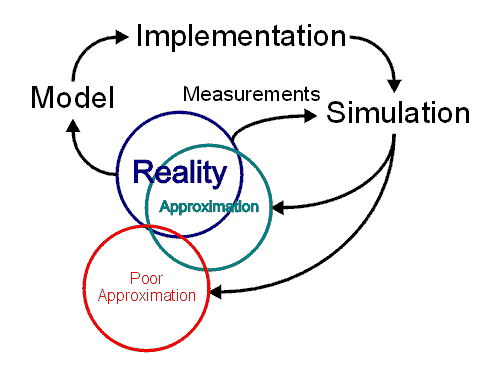## Introduction

The real world is exceedingly complex: one goal of an engineer is to be able to model or simulate the real world in such a way that the actual device need not be fabricated, but that the device may be simulated using computer programs.

Consider the example of the Airbus A380. This aircraft was modeled entirely on a computer before it was built, saving millions of dollars in research and development. Historically, any such development would require many physical models to be built and tested before the final design was ready. The accuracy of the models and the simulation are critical, for example, the weight savings resulting from removing the ashtrays in each seat in aircraft once smoking was banned on flights could not have been more than a few kilograms, however the suggestion earned the employee who gave it a very significant reward.

In developing circuit, the software SPICE takes a description of the circuit (you will see this in third year) and it will allow you to simulate the circuit on a PC. Thus, you may determine whether or not the circuit meets the given requirements based on the results of the simulation without ever building the circuit. This can save a lot of time and money in the research and development process.

The process of approximating the real world through simulation is given in Figure 1. It shows an overview of how the real world is approximated using mathematical models which are implemented using various algorithms on a computer which then take measurements from the real world to simulate the real world producing a (hopefully) appropriate approximation of the real world.Figure 1. The process of approximating the real world using numerical methods.

In the development of any device, initially, it is necessary to to first fabricate a device and after taking measurements, to determine an appropriate model which describes the behaviour of the device. After extracting the model, simulations are run with different inputs to determine that the model is appropriate. Once the model has been tested and approved, the model may be used for future development without first fabricating the device.

## The Model

A natural phenomenon is described by a mathematical model, usually by a differential equation. In some (simple) cases, these differential equations have exact solutions which we may use directly. In other cases, we must also solve these differential equations. In either case, we do this using algorithms implemented on a computer.

An engineer must use the most appropriate model, not the best model. For example, Einstein's general theory of relatively is the best model available to describe motion, but for some cases, Newton's laws of motion are more than sufficient, and for such cases, no employer will pay for a solution which has unnecessary precision. However, in others (for example, real-time conversion of optical Gbit/s signals to electric signals), Newton's laws no longer apply and it is necessary to use better models.

## Software Implementation

The mathematical models are then implemented in a program on a computer using various algorithms. This class covers many of the introductory algorithms used at this stage.

## Simulation

The executions of our simulations take measurements from the real world and produce an approximation of the real world. If we make judicious choices of the model, the algorithms used, and appropriate measurements, the approximation of the real world will be sufficiently good to satisfy the stated requirements. Errors in any of these steps can easily produce an invalid or poor representation of the real world which does not satisfy the given requirements.

# Sources of Error

There are a number of sources of error which may result in a poor approximation of the real world:

• Modeling Error: A wrong or inappropriate choice of model.
• Measurement Error: Incorrect or poor measurements.
• Implementation Error: Incorrect or poor choice of algorithms.
• Simulation Error: Error accumulated due to the execution of our model.

Of course, in any of these stages, you also have the human factor: a human may make a mistake due to carelessness or ignorance, most often as a result of measurement error.

We will see in this class how, due to the method which we choose to approximate real numbers on a computer, how error can accumulate during the simulation (or execution) of algorithms on a computer can lead to incorrect results. Hence, we will discuss appropriate algorithms which can be used to properly approximate our chosen models.

To do this, we will:

1. Define what we mean by error,
2. Look at the errors associated with approximating real numbers, and
3. Look at various algorithms which minimize this error, starting with appropriate means for solving systems of linear equations.